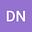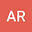Study on energy and information-entropic measures of Hulthén potential in D dimension by an intuitive approximation to centrifugal term
••Amlan Roy
Indian Institute of Science Education and Research Kolkata
Author Profile## Abstract

Energy spectrum as well as various information theoretic measures are considered for Hulthén potential in $$D$$ dimension. For a given $$\ell\neq 0$$ state, analytic expressions are derived, following a simple intuitive approximation for accurate representation of centrifugal term, within the conventional Nikiforov-Uvarov method. This is derived from a linear combination of two widely used Greene-Aldrich and Pekeris-type approximations. Energy, wave function, normalization constant, expectation value in $$r$$ and $$p$$ space, Heisenberg uncertainty relation, entropic moment of order $$\bar{\alpha}$$, Shannon entropy, Rényi entropy, disequilibrium, majorization as well as four selected complexity measures like LMC (López-Ruiz, Mancini, Calbert), shape Rényi complexity, Generalized Rényi complexity and Rényi complexity ratio are offered for different screening parameters ($$\delta$$). The effective potential is described quite satisfactorily throughout the whole domain. Obtained results are compared with theoretical energies available in literature, which shows excellent agreement. Performance of six different approximations to centrifugal term is critically discussed. An approximate analytical expression for critical screening for a specific state in arbitrary dimension is offered. Additionally, some inter-dimensional degeneracy occurring in two states, at different dimension for a particular $$\delta$$ is also uncovered.

PACS: 02.60.-x, 03.65.Ca, 03.65.Ge, 03.65.-w
Keywords: Hulthén potential, Rényi complexity ratio, Statistical complexity, Majorization, Pekeris approximation, Greene-Aldrich approximation.